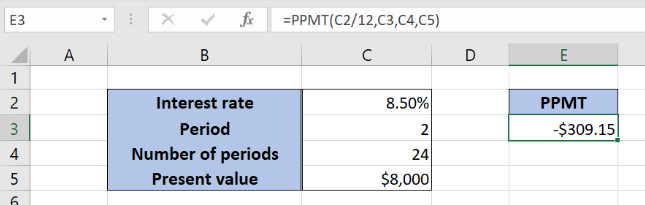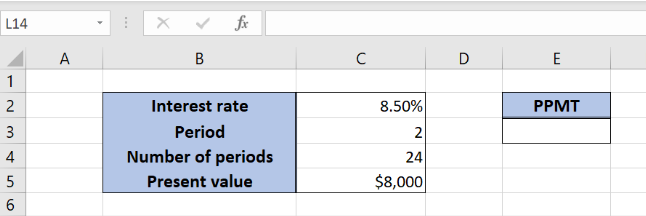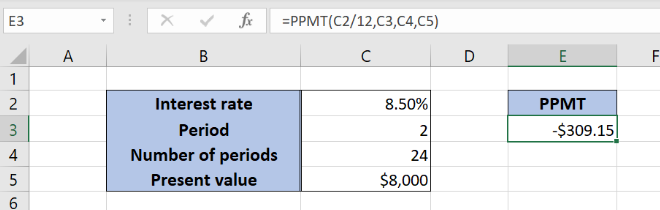Get instant live expert help with Excel or Google Sheets“My Excelchat expert helped me in less than 20 minutes, saving me what would have been 5 hours of work!”

#### Post your problem and you’ll get Expert help in seconds.

Your message must be at least 40 characters
Our professional Expert are available now. Your privacy is guaranteed.

# Learn How to Use the Excel PPMT Function

Excel allows a user to get a principal payment for any payment using the PPMT function. This step by step tutorial will assist all levels of Excel users in calculating a principal payment portion in a loan for a selected month.Figure 1. The result of the function

## Syntax of the PPMT Formula

The generic formula for the PPMT function is:

`=PPMT (rate, per, nper, pv, [fv], [type])`

The parameters of the PPMT function are:

• rate – an interest rate for the period (the annual rate divided by 12)
• per – a period for which we want to calculate a principal payment (must be between 1 and nper)
• nper – the total number of payments for a loan
• pv – the present value of a loan
• [fv] – the future value of a loan, after all payments. This is an optional parameter. If it’s omitted, the default value is 0
• [type] –  specifies when the payments are made: at the end of a period (0) or at the beginning of a period (1). By default, it’s 0.

## Setting up Our Data for the PPMT FunctionFigure 2. Data that we will use in the PPMT example

Let’s look at the structure of the data we will use. In the cell C2, we have the annual interest rate for the loan. In the cell C3 is the period for which we want to calculate the principal payment. In the cell C4 is the total number of payments, while in the C5 is the present value of the loan. We want to get the result of the PPMT function in the cell E3.

## Calculate the PPMT for the selected month

In our example, we want to get the principal payment for the second period in the cell E3. The interest rate is 8.50%, the total number of periods is 24, and the present value of the loan is \$8,000.Figure 3. Using the PPMT function to calculate the principal payment for the period

The formula looks like:

`=PPMT(C2/12, C3, C4, C5)`

The parameter rate is C2/12, as we must pass the monthly interest rate to the function. The per parameter is C3 (2), as we want to calculate the PPMT for the second period. The nper is the cell C4 (24), and the pv is in the C5 (\$8,000).

Finally, the result in the cell E3 is -\$309.15, which is the principal payment for the selected parameters. The result is always negative, as it is the payment.

To apply the PPMT function, we need to follow these steps:

• Select cell E3 and click on it
• Insert the formula: `=PPMT(C2/12, C3, C4, C5)`
• Press enter

Most of the time, the problem you will need to solve will be more complex than a simple application of a formula or function. If you want to save hours of research and frustration, try our live Excelchat service! Our Excel Experts are available 24/7 to answer any Excel question you may have. We guarantee a connection within 30 seconds and a customized solution within 20 minutes.